# RD Sharma Solutions Class 9 Chapter 24 Measure of Central Tendency

Read RD Sharma Solutions Class 9 Chapter 24 Measure of Central Tendency below, students should study RD Sharma class 9 Mathematics available on Studiestoday.com with solved questions and answers. These chapter wise answers for class 9 Mathematics have been prepared by teacher of Grade 9. These RD Sharma class 9 Solutions have been designed as per the latest NCERT syllabus for class 9 and if practiced thoroughly can help you to score good marks in standard 9 Mathematics class tests and examinations

Exercise 24.1

Question 1: If the heights of 5 persons are 140 cm, 150 cm, 152 cm, 158 cm and 161 cm respectively. Find the mean height.
Solution:
Sum of heights = 140 + 150 + 152 + 158 + 161 = 761
Total number of persons = 5
Mean height = (Sum of hieghts)/(Total Numbers of Person)
Mean height = 761/5
Mean height = 152.2
Hence, the mean height is 152.2.

Question 2: Find the mean of 994 , 996 , 998 , 1002 , 1000.
Solution:
Sum of numbers = 994 + 996 + 998 + 1000 + 1002 = 4990
Total counts of Numbers = 5
Mean = (Sum of numbers)/(Total Conts of Numbers)
Mean = 4990/5
Mean = 998
Hence, the mean of given numbers is 998.

Question 3: Find the mean of first five natural numbers.
Solution:
We know that,
1, 2, 3, 4, 5 are First five natural numbers.
Sum of all the numbers = 1 + 2 + 3 + 4 + 5 = 15
Total Numbers = 5
Mean = ((Sum of numbers))/((Total Numbers))
Mean = 15/5
Mean = 3
Hence, the mean of first five natural numbers are 3.

Question 4: Find the mean of all factors of 10.
Solution:
We know that,
Factors of 10 are 1, 2, 5, 10
Sum of all the factors = 1+2+5+10 = 18
Total Numbers = 4
Mean = (Sum of factors)/(Total Numbers)
Mean = 18/4
Mean = 4.5
Hence, the mean of all factors of 10 is 4.5.

Question 5: Find the mean of first 10 even natural numbers.
Solution:
We know that,
First 10 even natural numbers = 2 , 4 , 6 , 8 , 10 , 12 , 14 , 16 , 18 , 20
Sum of numbers = 2 + 4 + 6 + 8 + 10 + 12 + 14 + 16 + 18 + 20 = 110
Total Numbers = 10
Now,
Mean = (Sum of numbers)/(Total Numbers)
=  110/10
Mean = 11
Hence, the mean of first 10 even natural numbers is 11.

Question 6: Find the mean of x , x + 2 , x + 4 , x + 6 , x + 8.
Solution:
It is given that, the numbers are x, x + 2, x + 4, x + 6, x + 8
Sum of numbers = x + (x+2) + (x+4) + (x+6) + (x+8) = 5x + 20
Total Numbers = 5
Mean = (Sum of numbers)/(Total Numbers)
Mean = ((5x+20))/5
Mean =  (5(x+4))/5
Mean = x + 4
Mean = x + 4
Hence, the Mean of given data is x + 4.

Question 7: Find the mean of first five multiples of 3.
Solution:
3, 6, 9, 12, 15 are the first five multiples of 3.
Sum of numbers = 3 + 6 + 9 + 12 + 15 = 45
Total Numbers = 5
Mean = (Sum of numbers)/(Total Numbers)
Mean = 45/5
Mean = 9
Hence, 9 is the mean of first five multiples of 3.

Question 8: Following are the weights (in kg) of 10 new born babies in a hospital on a particular day: 3.4 , 3 .6 , 4.2 , 4.5 , 3.9 , 4.1 , 3.8 , 4.5 , 4.4 , 3.6. Find the mean.
Solution:
Sum of weights = 3.4 + 3.6 + 4.2 + 4.5 + 3.9 + 4.1 + 3.8 + 4.5 + 4.4 + 3.6 = 40
Total number of babies = 10
Mean = (Sum of weights)/(Total number of babies)
Mean = 40/10
Mean = 4
Hence, the mean weight of new born babies is 4 kg

Question 9: The percentage marks obtained by students of a class in mathematics are :
64 , 36 , 47 , 23 , 0 , 19 , 81 , 93 , 72 , 35 , 3 , 1. Find their mean.
Solution:
Sum of marks = 64 + 36 + 47 + 23 + 0 + 19 + 81 + 93 + 72 + 35 + 3 + 1 = 474
Total students = 12
Mean = (Sum of marks)/(Total students)
Mean = 274/12
Mean = 39.5
Hence, the mean mark in mathematics is 39.5.

Question 10: The numbers of children in 10 families of a locality are:
2 , 4 , 3 , 4 , 2 , 3 , 5 , 1 , 1 , 5. Find the number of children per family.
Solution:
Total number of children = 2 + 4 + 3 + 4 + 2 + 3 + 5 + 1 + 1 + 5 = 30
Total Families = 10
Number of children per family =
Mean = (Total number of children )/(Total Families)
Mean = 30/10
Mean = 3
Hence, Number of children per family is 3.

Exercise 24.2

Question 1: Calculate the mean for the following distribution: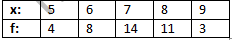Solution: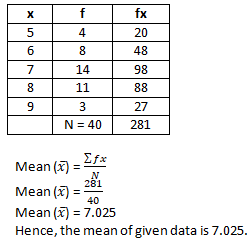Question 2: Find the mean of the following data:Solution:Question 3: The mean of the following data is 20.6 .Find the value of p.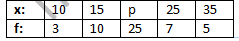Solution:Question 4: If the mean of the following data is 15, find p.Solution: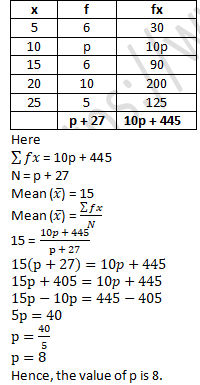Question 5: Find the value of p for the following distribution whose mean is 16.6.Solution:Question 6: Find the missing value of p for the following distribution whose mean is 12.58.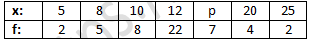Solution: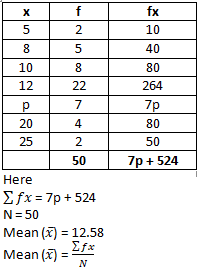12.58 = (7p + 524)/50
12.58×50=7p+524
629=7p+524
629-524=7p
105=7p
-7p=-524+629
-7p=-105
p=(-105)/(-7)
p=15
Hence, the value of p is 15.

Question 7: Find the missing frequency (p) for the following distribution whose mean is 7.68.Solution: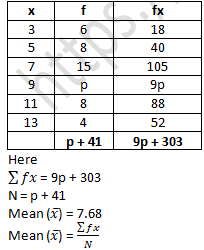7.68 = (9p + 303)/(p + 41)
7.68(p+41)=9p + 303
7.68p + 314.88 = 9p + 303
7.68p - 9p = 303 - 314.88
-1.32p = -11.88
p = (-11.88)/(-1.32)
Hence, the value of p is 9.

Exercise 24.3

Question 1: Find the median of the following data:
83 , 37 , 70 , 29 , 45 , 63 , 41 , 70 , 34 , 54
Solution:
Arranging the date in ascending order: 29, 34, 37, 41, 45, 54, 63, 70, 70, 83
Total number of terms (N) = 10
Here N is even,
Median = (n/2 th term + (n/2+1)th term)/2
Median = (10/2 th term + (10/2+1)th term)/2
Median = (5^th  term + 6^th  term)/2
Median = (45 + 54)/2
Median = 99/2
Median = 49.5
Hence, the median is 49.5.

Question 2: Find the median of the following data:
133 , 73 , 89 , 108 , 94 , 104 , 94 , 85 , 100 , 120
Solution:
Arranging the date in ascending order: 73, 85, 89, 94, 94, 100, 104, 108, 120, 133.
Total number of terms (N) = 10
Here N is even,
Median = (n/2 th term + (n/2+1)th term)/2
Median = (10/2 th term + (10/2+1)th term)/2
Median = (5th  term + 6th  term)/2
Median = (94 + 100)/2
Median = 194/2
Median = 97
Hence, the median is 97.

Question 3: Find the median of the following data:
31, 38, 27, 28, 36, 25, 35, 40
Solution:
Arranging the date in ascending order: 25, 27, 28, 31, 35, 36, 38, 40.
Total number of terms (N) = 8
Here N is even,Question 4: Find the median of the following data:
15, 6, 16, 8, 22, 21, 9, 18, 25
Solution:
Arranging the date in ascending order: 6, 8, 9, 15, 16, 18, 21, 22, 25
Total number of terms (N) = 9
Median = ((n+1)/2)th term
Median = ((9+1)/2)th term
Median = (10/2)th term
Median = 5th  term
Here 5th term is 16.
Hence, the median is 16.

Question 5: Find the median of the following data:
41, 43, 127, 99, 71, 92, 71, 58, 57
Solution:
Arranging the date in ascending order: 41, 43, 57, 58, 71, 71, 92, 99, 127
Total number of terms (N) = 9
Here N is odd,
Median = ((9+1)/2)th term
Median = (10/2)th term
Median = 5th  term
Here 5th term is 71.
Hence, the median is 71.

Question 6: Find the median of the following data:
25, 34, 31, 23, 22, 26, 35, 29, 20, 32
Solution:
Arranging the date in ascending order: 20, 22, 23, 25, 26, 29, 31, 32, 34, 35
Total number of terms (N) = 10
Here N is even,Question 7: Find the median of the following data:
12, 17, 3, 14, 5, 8, 7, 15
Solution:
Arranging the date in ascending order: 3, 5, 7, 8, 12, 14, 15, 17
Total number of terms (N) = 8
Here N is even,Question 8: Find the median of the following data:
92 , 35 , 67 , 85 , 72 , 81 , 56 , 51 , 42 , 69
Solution:
Arranging given numbers in ascending order
35 , 42 , 51 , 56 , 67 , 69 , 72 , 81 , 85 , 92
Here N is even,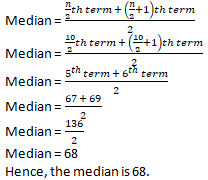Exercise 24.4

Question 1: Find out the mode of the following marks obtained by 15 students in a class:
Marks : 4 , 6 , 5 , 7 , 9 , 8 , 10 , 4 , 7 , 6 , 5 , 9 , 8 , 7 , 7.
Solution:
The most common value in a series of observations is called the mode.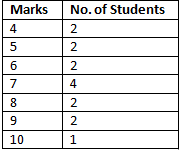Here, 7 occurred most frequently.
Hence, Mode of the given data is 7.

Question 2: Find out the mode from the following data:
125, 175, 225, 125, 225, 175, 325, 125, 375, 225, 125
Solution:
Find the frequency of given set of observations: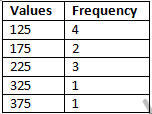Here 125 occurred most frequently.
Hence, Mode of the given data is 125.

Question 3: Find the mode for the following series:
7.5 , 7.3 , 7.2 , 7.2 , 7.4 , 7.7 , 7.7 , 7.5 , 7.3 , 7.2 , 7.6 , 7.2
Solution: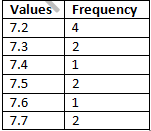Here 7.2 occurred most frequently.
Hence, Mode of the given data is 7.2.

Exercise VSAQs

Question 1: If the ratio of mean and median of a certain data is 2:3, then find the ratio of its mode and mean.
Solution:
Let us assumed that,
Mean = 2x
Median = 3x
Mode = 3Median – 2Mean
Mode = 3(3x) – 2(2x)
Mode = 9x – 4x
Mode = 5x
Mode: Mean = 5x : 2x
Hence, the ratio of Mode to Mean is 5: 2.

Question 2: If the ratio of mode and median of a certain data is 6 : 5, then find the ratio of its mean and median.
Solution:
It is given that, the ratio of mode and median is 6 : 5
Mode/Median = 6/5
By cross-multiply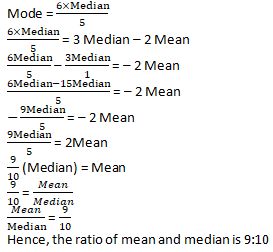Question 3: If the mean of x+2, 2x+3, 3x+4, 4x+5 is x+2, find x.
Solution:
It is given that,
Mean = x+2
And Observations x+2, 2x+3, 3x+4, 4x+5
Mean = (Sum of all the observations)/(Total number of observations)
Mean = (x+2 + 2x+3 + 3x+4 + 4x+5)/4
Mean = ((10x + 14))/4
(x + 2) = ((10x + 14))/4
4(x+2) =(10x+14)
4x+8 = 10x+14
x=-1
Hence, the value of x is -1.

Question 4: The arithmetic mean and mode of a data are 24 and 12 respectively, then find the median of the data.
Solution:
It is given that,
The arithmetic mean = 24
Mode = 12
Mode = 3Median – 2Mean
3Median = Mode + 2Mean
3Median = 12 + 2(24)
3Median = 60
Median = 60/3
Median = 20
Hence, the median is 20.

Question 5: If the difference of mode and median of a data is 24, then find the difference of median and mean.
Solution:
It is given that,
Mode – Median = 24
Mode = 24 + Median
Mode = 3 Median – 2 Mean
24 + Median = 3Median – 2Mean
24 = 2Median – 2Mean
12 = Median – Mean
Hence, the difference of median and mean is 12.